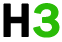CSharp基础 专题

C#变量

c# 中的每个变量都有一个特定的类型，它决定了变量内存的大小和布局，可以存储在该存储器中的值的范围以及可应用于该变量的一组操作。

c# 中提供的基本值类型可以分为：

c# 还允许定义变量的其他值类型，例如：枚举和引用类型的变量，如类，我们将在后续章节中介绍。

## 定义变量

c# 中变量定义的语法是：

``````data_type variable_list;
``````

``````int i, j, k;
char c, ch;
float f, salary;
double d;
``````

``````int i = 1999;
``````

## 初始化变量

``````variable_name = value;
``````

``````<data_type> <variable_name> = value;
``````

``````int d = 3, f = 5;    /* initializing d and f. */
byte z = 22;         /* initializes z. */
double pi = 3.14159; /* declares an approximation of pi. */
char x = 'x';        /* the variable x has the value 'x'. */
``````

``````using system;
namespace variabledefinition
{
class program
{
static void main(string[] args)
{
short a;
int b ;
double c;

/* actual initialization */
a = 10;
b = 20;
c = a + b;
console.writeline("a = {0}, b = {1}, c = {2}", a, b, c);
}
}
}
``````

``````a = 10, b = 20, c = 30
``````

## 从用户接受值

`system`命名空间中的`console`类提供了一个用于接受用户输入并将其存储到变量中的`readline()`函数。

``````int num;
``````

## c# 中的左值和右值表达式：

c# 中有两种表达式：

• 左值(`lvalue`)：一个左值或右值的表达式可表示为左值或右侧。
• 右值(`rvalue`)：一个右值表达式可出现在赋值的右侧，而不是左侧。

``````int g = 20;
``````

``````10 = 20;
``````

## 定义变量的规则

• 一个变量可以有字母，数字和下划线。
• 变量名称只能以字母和下划线开头。
• 它不能以数字开头。
• 变量名称内不允许有空格。
• 变量名称不能是任何保留字或关键词。如：`char``float`

``````int x;
int _x;
int k20;
``````

``````int 4;
int x y;
int double;
``````